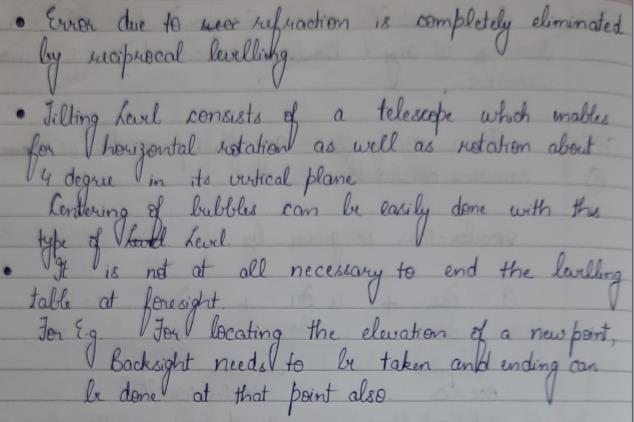Courses

# Test: Levelling - 1

## 10 Questions MCQ Test GATE Civil Engineering (CE) 2022 Mock Test Series | Test: Levelling - 1

Description
This mock test of Test: Levelling - 1 for Civil Engineering (CE) helps you for every Civil Engineering (CE) entrance exam. This contains 10 Multiple Choice Questions for Civil Engineering (CE) Test: Levelling - 1 (mcq) to study with solutions a complete question bank. The solved questions answers in this Test: Levelling - 1 quiz give you a good mix of easy questions and tough questions. Civil Engineering (CE) students definitely take this Test: Levelling - 1 exercise for a better result in the exam. You can find other Test: Levelling - 1 extra questions, long questions & short questions for Civil Engineering (CE) on EduRev as well by searching above.
QUESTION: 1

### A ‘level line’ is a

Solution:

A level line is the distance between 2 points on the level surface, which follows the curvature of the earth. Whereas the line of sight is the straight line perpendicular to the plumb line at the telescope.

QUESTION: 2

Solution:
QUESTION: 3

### With the rise of temperature, the sensitivity of a bubble tube

Solution:

With a rise in temperature the liquid expands. Hence the bubble shortens and consequently its sensitivity decreases.

QUESTION: 4

As applied to staff readings, the corrections for curvature and refraction are respectively

Solution:
QUESTION: 5

Which of the following arithmetic checks can be applied in rise and fall method?

Solution:
QUESTION: 6

The correction for refraction as applied to staff reading is
where R is radius of earth

Solution:

Correction due to refraction is approximately about 1/7th of correction due to earth curvature.
CR = 1/7 Cc
= 1/7[d2/2R]

QUESTION: 7

While doing levelling in undulating terrain, it is preferable to set the level on

Solution:
QUESTION: 8

Which of the following statements is incorrect?

Solution:QUESTION: 9

Benchmark is established by

Solution:
QUESTION: 10

The curvature of the earth’s surface, is taken into account only if the extent of survey is more than

Solution:

Geodetic Survey is the survey in which earth’s curvature is taken into account for calculation. It is generally done when area to be covered is more than 260 km2